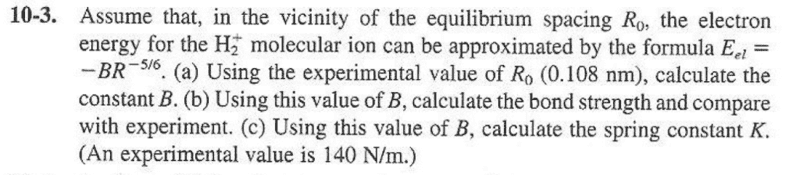# Bond Strength and Force constant

Muthumanimaran

## Homework Statement## The Attempt at a Solution

(a) Total energy is
$$E=\frac{-e^2}{4\pi\epsilon_{0}R}-\frac{B}{R^{5/6}}$$

Taking derivative of E with respect to R and equating it to zero when R=R0 yields,
i.e,
$$\frac{dE}{dR}{\bigg|}_{R=R_0}=0$$
when R=R0
yields,
$$B=\frac{3e^2}{10\pi\epsilon_{0}{R_{0}}^{1/6}}$$

How to proceed with part (b) and part (c)? giving me hints to solve this problem is highly helpful.